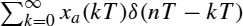# Frequency-Domain Analysis

## 3.1 INTRODUCTION

In the previous chapter, we derived the definition for the z transform of a discrete-time signal by impulse-sampling a continuous-time signal xa(t) with a sampling period T and using the transformation z = esT. The signal xa(t) has another equivalent representation in the form of its Fourier transform X(). It contains the same amount of information as xa(t) because we can obtain xa(t) from X() as the inverse Fourier transform of X(). When the signal xa(t) is sampled with a sampling period T, to generate the discrete-time signal represented by, the following questions need to be answered:

Is there an equivalent representation for the discrete-time signal in the frequency domain?

Does it contain the same amount of information as that found in xa(t)? If so, how do we reconstruct xa(t) from its sample values xa(nT)?

Does the Fourier transform represent the frequency response of the system when the unit impulse response h(t) of the continuous-time system is sampled? Can we choose any value for the sampling period, or is there a limit that is determined by the input signal or any other considerations?

We address these questions in this chapter, arrive at the definition for the discrete-time Fourier transform (DTFT) of the discrete-time system, and describe its properties and applications. In the second half of the chapter, we ...

Get Introduction to Digital Signal Processing and Filter Design now with the O’Reilly learning platform.

O’Reilly members experience books, live events, courses curated by job role, and more from O’Reilly and nearly 200 top publishers.